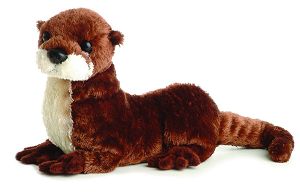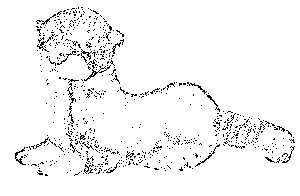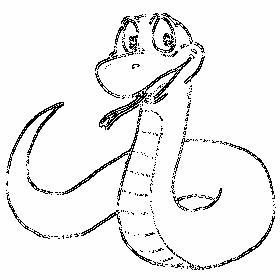# John Lester Image Portfolio

Lab 1 | Lab 2 | Lab 3 | Lab 4 | Lab 5 | Lab 6 | Lab 7 | Bonus

 Lab 1: Digital Media Creation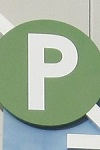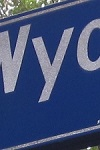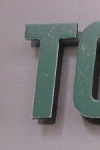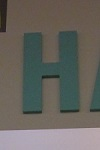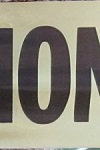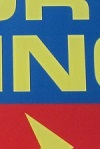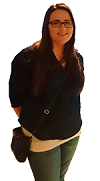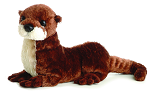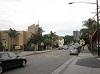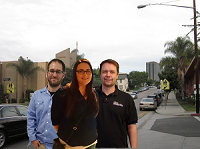Lab 2: Getting Started with JES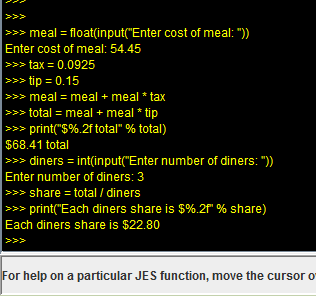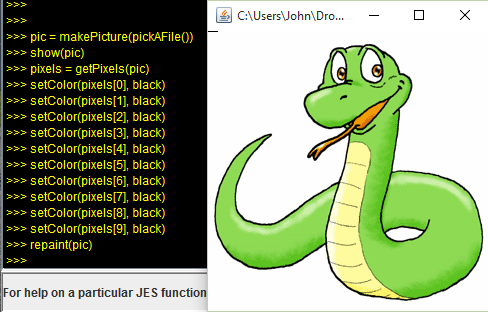Lab 3: Manipulating Images with Python # CST205 Lab 3 - Programmer: John Lester pic = makePicture(pickAFile()) show(pic) def halfRed(pic = pic): pic = lessRed(50) return pic def noBlue(pic = pic): pixels = getPixels(pic) for pix in pixels: setBlue(pix, 0) repaint(pic) return pic def lessRed(amount, pic = pic): pixels = getPixels(pic) for pix in pixels: r = getRed(pix) setRed(pix, r - r * amount * 0.01) repaint(pic) return pic def moreRed(amount, pic = pic): pixels = getPixels(pic) for pix in pixels: r = getRed(pix) mr = r + r * amount * 0.01 if mr >= 255: setRed(pix, 255) else: setRed(pix, mr) repaint(pic) return pic def roseColoredGlasses(pic = pic): pixels = getPixels(pic) for pix in pixels: r = getRed(pix) g = getGreen(pix) b = getBlue(pix) if r <= 212: setRed(pix, r * 1.2) else: setRed(pix, 255) setGreen(pix, g * 0.4) setBlue(pix, b * 0.8) repaint(pic) return pic def lightenUp(pic = pic): pixels = getPixels(pic) for pix in pixels: c = getColor(pix) nc = makeLighter(c) setColor(pix, nc) repaint(pic) return pic def makeNegative(pic = pic): pixels = getPixels(pic) for pix in pixels: r = getRed(pix) g = getGreen(pix) b = getBlue(pix) setRed(pix, 255 - r) setGreen(pix, 255 - g) setBlue(pix, 255 - b) repaint(pic) return pic def BnW(pic = pic): pixels = getPixels(pic) for pix in pixels: r = getRed(pix) g = getGreen(pix) b = getBlue(pix) intensity = int((r + g + b) / 3) setRed(pix, intensity) setGreen(pix, intensity) setBlue(pix, intensity) repaint(pic) return pic def betterBnW(pic = pic): pixels = getPixels(pic) for pix in pixels: r = getRed(pix) g = getGreen(pix) b = getBlue(pix) intensity = (r * 0.299 + g * 0.587 + b * 0.114) setRed(pix, intensity) setGreen(pix, intensity) setBlue(pix, intensity) repaint(pic) return pic pic = BnW()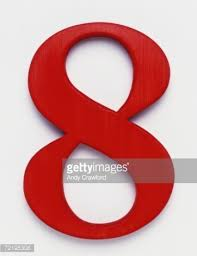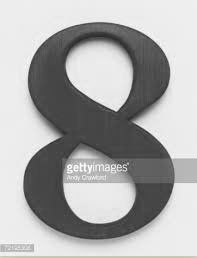pic = halfRed()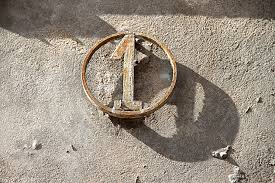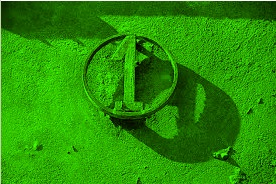pic = noBlue()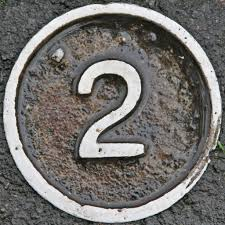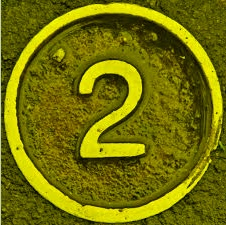pic = lessRed(100)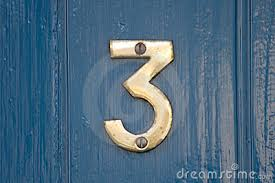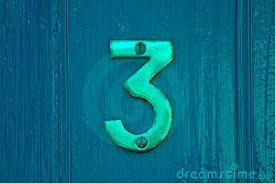pic = moreRed(100)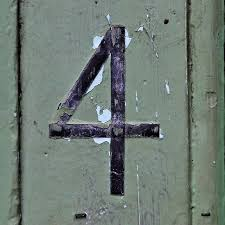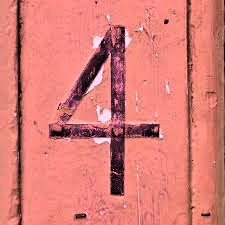pic = roseColoredGlasses() For this function we parse each pixel and add 20% to the red but reduce green by 60% and blue by 20%.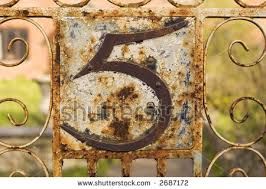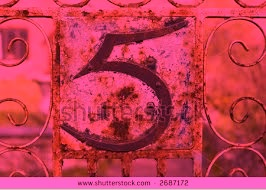pic = lightenUp()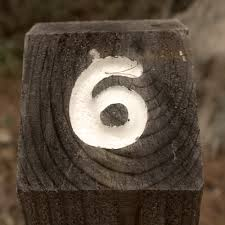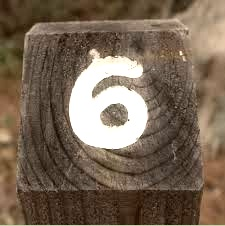pic = makeNegative() For this function we again parse each pixel but take each red, green and blue values and subtract them from 255 to get the inverse.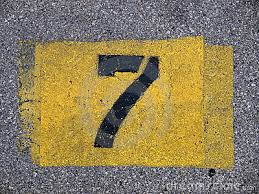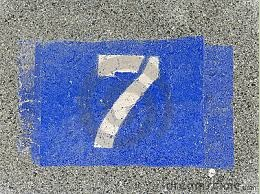pic = betterBnW() For this function we again parse each pixel and reset their RGB values to (r * 0.299 + g * 0.587 + b * 0.114) to produce gray tones.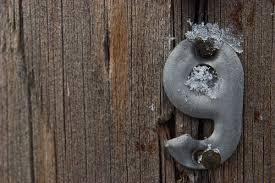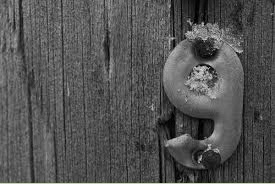Lab 4: Modifying pictures pixel by pixel # CST205 Lab 4 - Programmer: John Lester pic = makePicture(pickAFile()) show(pic) def halfBetter(pic = pic): for x in range(getWidth(pic)/2, getWidth(pic)): for y in range(0, getHeight(pic)): pix = getPixel(pic, x, y) r = getRed(pix) g = getGreen(pix) b = getBlue(pix) intensity = (r * 0.299 + g * 0.587 + b * 0.114) setRed(pix, intensity) setGreen(pix, intensity) setBlue(pix, intensity) repaint(pic) return pic def leftMirror(pic = pic): for x in range(0, getWidth(pic)/2): for y in range(0, getHeight(pic)): pix = getPixel(pic, x, y) r = getRed(pix) g = getGreen(pix) b = getBlue(pix) newPix = getPixel(pic, getWidth(pic)-1-x, y) setRed(newPix, r) setGreen(newPix, g) setBlue(newPix, b) repaint(pic) return pic def rightMirror(pic = pic): for x in range(getWidth(pic)/2, getWidth(pic)): for y in range(0, getHeight(pic)): pix = getPixel(pic, x, y) r = getRed(pix) g = getGreen(pix) b = getBlue(pix) newPix = getPixel(pic, getWidth(pic)-1-x, y) setRed(newPix, r) setGreen(newPix, g) setBlue(newPix, b) repaint(pic) return pic def topMirror(pic = pic): for x in range(0, getWidth(pic)): for y in range(0, getHeight(pic)/2): pix = getPixel(pic, x, y) r = getRed(pix) g = getGreen(pix) b = getBlue(pix) newPix = getPixel(pic, x, getHeight(pic)-1-y) setRed(newPix, r) setGreen(newPix, g) setBlue(newPix, b) repaint(pic) return pic def bottomMirror(pic = pic): for x in range(0, getWidth(pic)): for y in range(getHeight(pic)/2, getHeight(pic)): pix = getPixel(pic, x, y) r = getRed(pix) g = getGreen(pix) b = getBlue(pix) newPix = getPixel(pic, x, getHeight(pic)-1-y) setRed(newPix, r) setGreen(newPix, g) setBlue(newPix, b) repaint(pic) return pic def simpleCopy(pic = pic): newPic = makeEmptyPicture(getWidth(pic), getHeight(pic)) for x in range(0, getWidth(pic)): for y in range(0, getHeight(pic)): pix = getPixel(pic, x, y) r = getRed(pix) g = getGreen(pix) b = getBlue(pix) newPix = getPixel(newPic, x, y) setRed(newPix, r) setGreen(newPix, g) setBlue(newPix, b) show(newPic) return newPic def rotatePic(pic = pic): newPic = makeEmptyPicture(getHeight(pic), getWidth(pic)) for x in range(0, getWidth(pic)): for y in range(0, getHeight(pic)): pix = getPixel(pic, x, y) r = getRed(pix) g = getGreen(pix) b = getBlue(pix) newPix = getPixel(newPic, y, x) setRed(newPix, r) setGreen(newPix, g) setBlue(newPix, b) repaint(newPic) return newPic def reducePic(pic = pic): newPic = makeEmptyPicture(getHeight(pic)/2, getWidth(pic)/2) for x in range(0, getWidth(pic), 2): for y in range(0, getHeight(pic), 2): pix = getPixel(pic, x, y) r = getRed(pix) g = getGreen(pix) b = getBlue(pix) newPix = getPixel(newPic, x/2, y/2) setRed(newPix, r) setGreen(newPix, g) setBlue(newPix, b) repaint(newPic) return newPic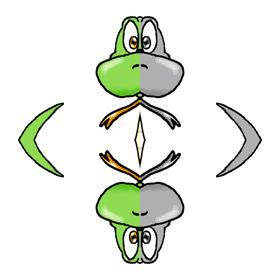pic = halfBetter()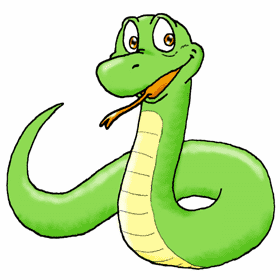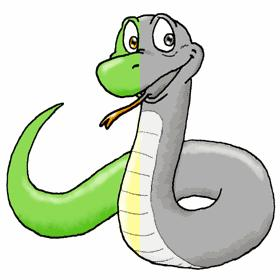pic = leftMirror()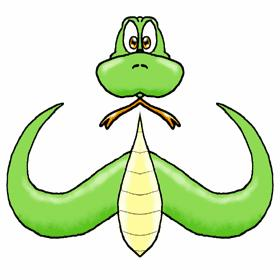pic = rightMirror()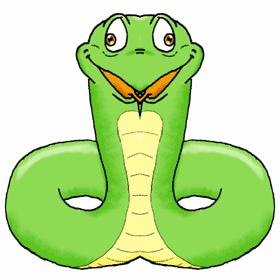pic = topMirror()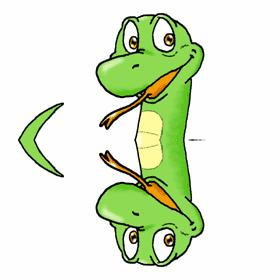pic = bottomMirror() For this function we copy the bottom half by limiting y to the middle and down then copy them to the upper half.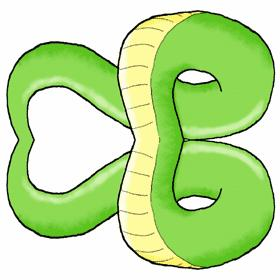pic = rotatePic()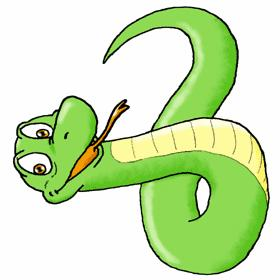pic = reducePic() This function reduces the image in half by creating an image half as large and copying every other pixel over to it.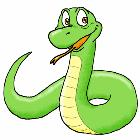Lab 5: Advanced image manipulation # CST205 Lab 5 - Programmer: John Lester def pyCopy(source, target, targetX, targetY): pic = makePicture(source) newPic = makePicture(target) for x in range(0, getWidth(pic)): if x + targetX < getWidth(newPic): for y in range(0, getHeight(pic)): if y + targetY < getHeight(newPic): pix = getPixel(pic, x, y) r = getRed(pix) g = getGreen(pix) b = getBlue(pix) if r != 0 and g != 215 and b != 0: # dont copy chroma key backgrounds newPix = getPixel(newPic, x + targetX, y + targetY) setRed(newPix, r) setGreen(newPix, g) setBlue(newPix, b) writePictureTo(newPic, target) return newPic For this lab we took various pictures and applied filters from earlier labs. Then we put them together into a larger collage image.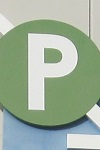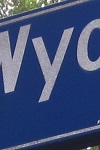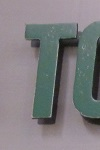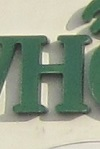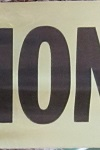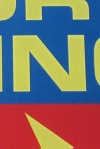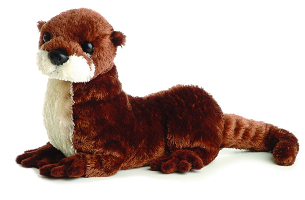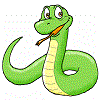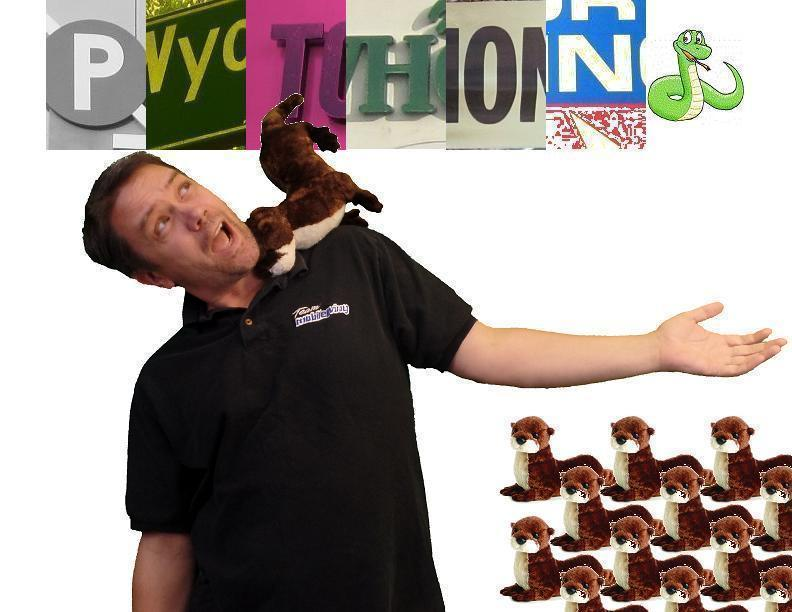Lab 6: Changing regions of pictures # CST205 Lab 6 - Programmer: John Lester pic = makePicture(pickAFile()) show(pic) def redEyeRabbit(newColor, pic = pic): for x in range(780, 1000): # specific to image for y in range(250, 470): # specific to image px = getPixel(pic, x, y) color = getColor(px) # even color selection is specific to this image if (distance(color, Color(211, 87, 106)) < 50) or (distance(color, Color(238, 153, 129)) < 50): setColor(px, newColor) if (distance(color, Color(255, 249, 160)) < 50) or (distance(color, Color(248, 214, 95)) < 50): setColor(px, newColor) show(pic) return pic def sepia(pic = pic): pixels = getPixels(pic) for pix in pixels: r = getRed(pix) g = getGreen(pix) b = getBlue(pix) intensity = (r * 0.299 + g * 0.587 + b * 0.114) r = intensity g = intensity b = intensity if r < 63: r = r * 1.1 b = b * 0.9 elif r < 192: r = r * 1.15 b = b * 0.85 else: if r * 1.08 > 255: r = 255 else: r = r * 1.08 b = b * 0.93 setRed(pix, r) setGreen(pix, g) show(pic) return pic def Artify(pic = pic): pixels = getPixels(pic) for pix in pixels: r = getRed(pix) g = getGreen(pix) b = getBlue(pix) if r < 64: setRed(pix, 31) elif r < 128: setRed(pix, 95) elif r < 192: setRed(pix, 159) else: setRed(pix, 223) if g < 64: setGreen(pix, 31) elif g < 128: setGreen(pix, 95) elif g < 192: setGreen(pix, 159) else: setGreen(pix, 223) if b < 64: setBlue(pix, 31) elif b < 128: setBlue(pix, 95) elif b < 192: setBlue(pix, 159) else: setBlue(pix, 223) show(pic) return pic def chromakey(addition, targetFile, targetX, targetY, overRide = False): newPic = makePicture(addition) bckPic = makePicture(targetFile) for x in range(0, getWidth(newPic)): if x + targetX < getWidth(bckPic): for y in range(0, getHeight(newPic)): if y + targetY < getHeight(bckPic): pix = getPixel(newPic, x, y) bPix = getPixel(bckPic, x + targetX, y + targetY) color = getColor(pix) bColor = getColor(bPix) if (distance(color, Color(0, 215, 0)) > 50) and ((distance(bColor, Color(0, 215, 0)) < 60) or (distance(bColor, Color(0, 131, 0)) < 60) or (distance(bColor, Color(45, 221, 45)) < 60) or overRide): newPix = getPixel(bckPic, x + targetX, y + targetY) setColor(bPix, color) writePictureTo(bckPic, targetFile) show(bckPic) return bckPic pic = redEyeRabbit(blue) For this function we built a custom routine for a particular image. For more advanced purposes it would be better to find a way to detect red eye.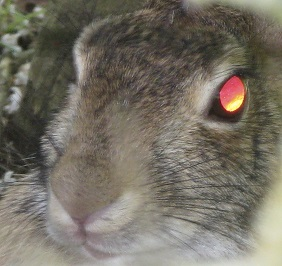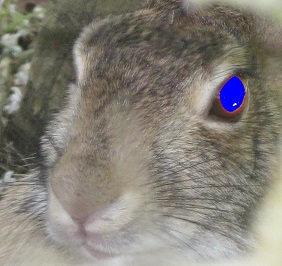pic = sepia()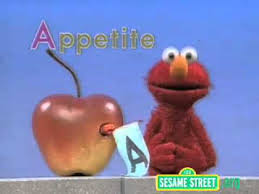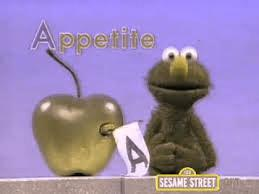pic = Artify() For this function we limit the RGB values of each pixel to 4 values - 31, 95, 159 and 223.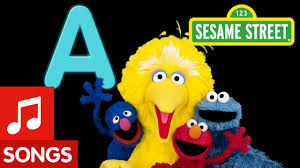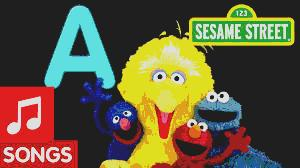pic = chromakey(alien, apolloReal, 500, 50) This was perhaps the funnest lab so far. We took two images, x-y coordinates and a boolean override input to merge. The first variable was the addition, next was the background, then x-y coordinates to place addition. The default behavior is to only copy non-green on green areas, but the boolean override says to print all non-green onto all areas.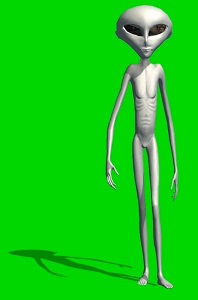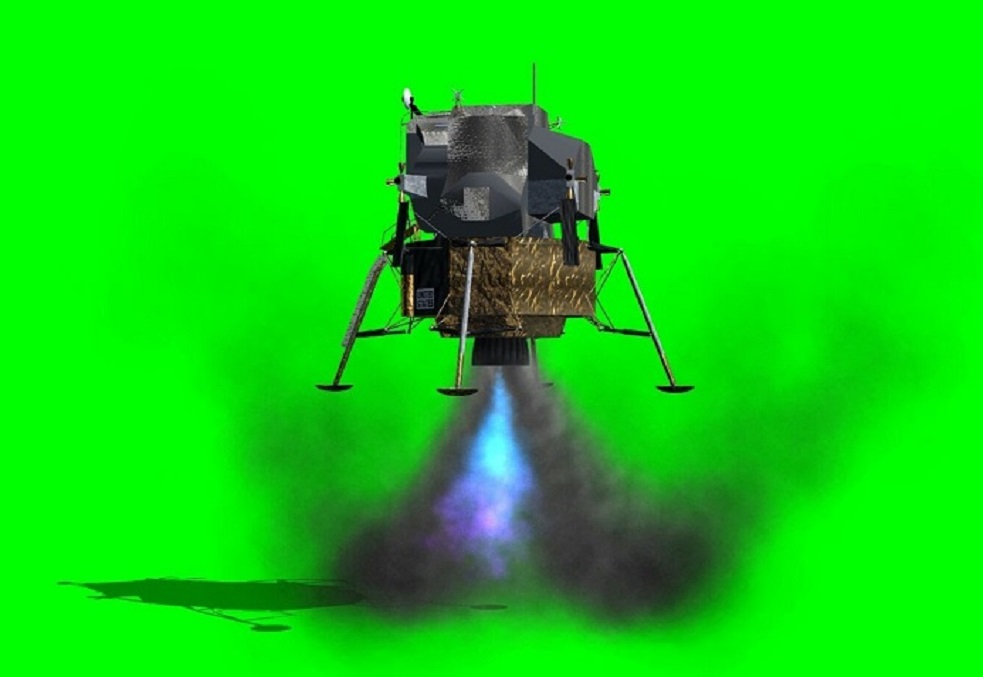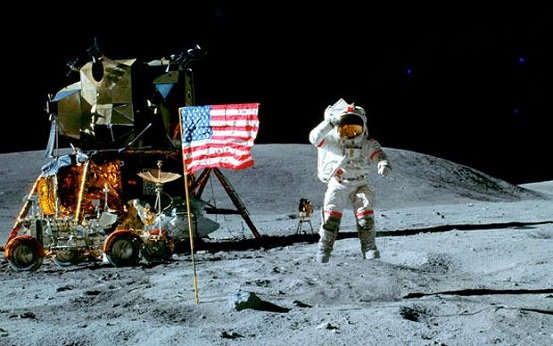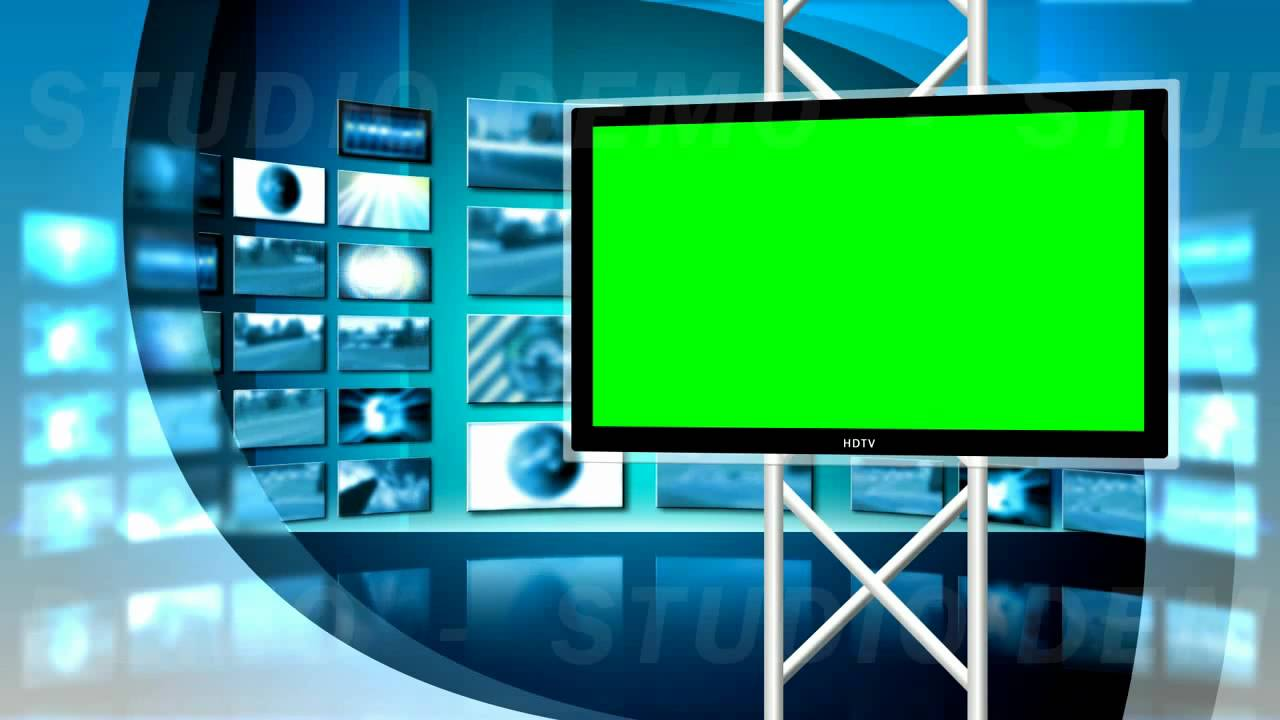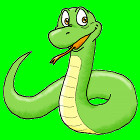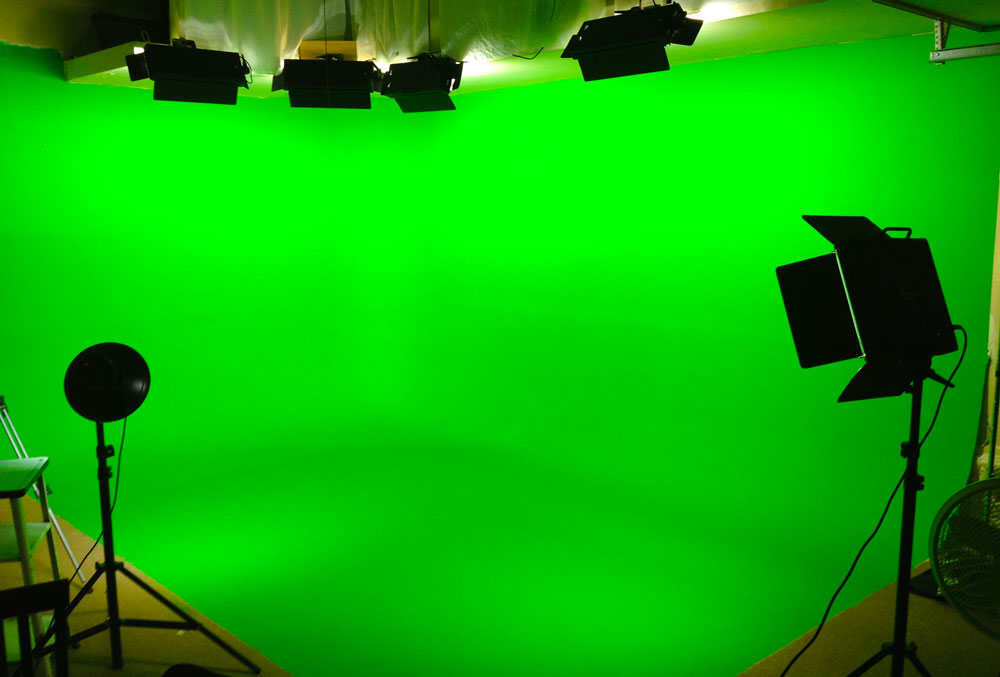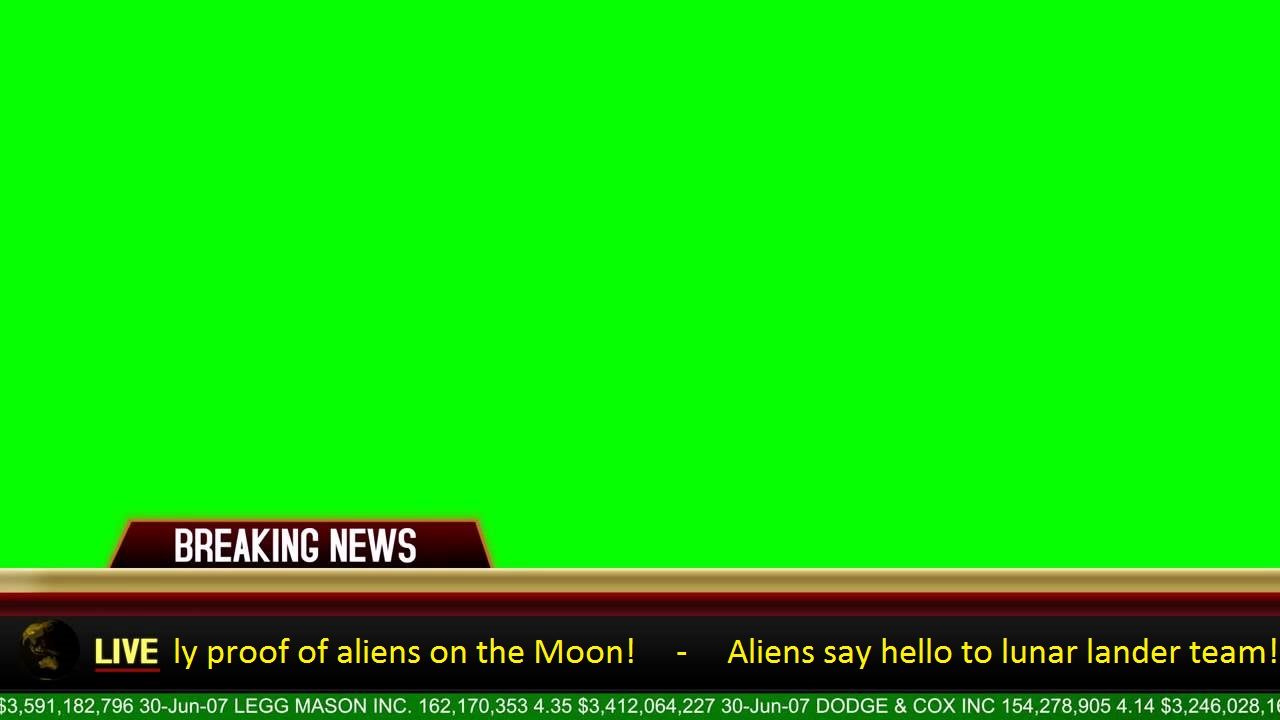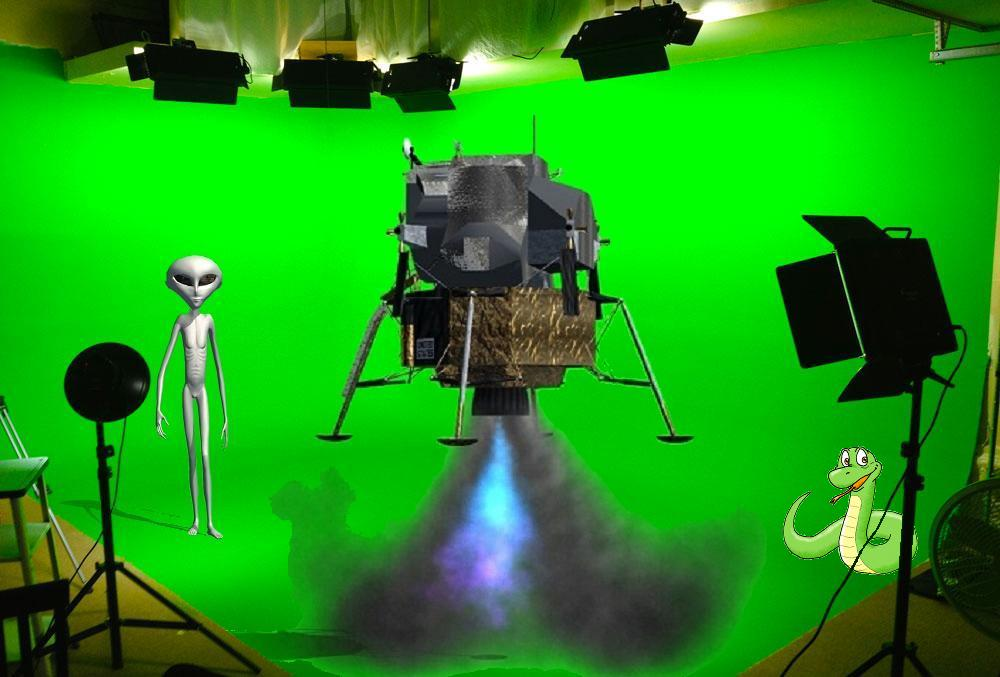Lab 7: Homemade Thanksgiving # CST205 Lab 7 - Programmer: John Lester # Welcome to the BITsoft Thanksgiving card generator v1.0 # Use pic = makeCard() to pick your background, then insert picture, then CSUMB logo # Use writePictureTo(pic, 'C:\\Path\\To\\Save\\image.jpg') to save result def chromakey(newPic, bckPic, targetX = 0, targetY = 0, overRide = False, tolerance = 60): for x in range(0, getWidth(newPic)): if x + targetX < getWidth(bckPic): for y in range(0, getHeight(newPic)): if y + targetY < getHeight(bckPic): pix = getPixel(newPic, x, y) bPix = getPixel(bckPic, x + targetX, y + targetY) color = getColor(pix) bColor = getColor(bPix) if (distance(color, Color(0, 215, 0)) > tolerance) and ((distance(bColor, Color(0, 215, 0)) < 60) or (distance(bColor, Color(0, 131, 0)) < 60) or (distance(bColor, Color(45, 221, 45)) < 60) or overRide): newPix = getPixel(bckPic, x + targetX, y + targetY) setColor(bPix, color) return bckPic def shrink(origPic, factor): if factor <= 1: return origPic width = getWidth(origPic) height = getHeight(origPic) newPic = makeEmptyPicture(int(width / factor), int(height / factor)) oldX = 0 for newX in range(0, getWidth(newPic)): oldY = 0 for newY in range(0, getHeight(newPic)): oldPix = getPixel(origPic, oldX, oldY) newPix = getPixel(newPic, newX, newY) setColor(newPix, getColor(oldPix)) oldY += factor oldX += factor return newPic def addThxMessage(pic, text): size = 48 #centering only kinda works with 48pt text xPos = int((getWidth(pic)/2) - (len(text) * 12.6)) yPos = size style = makeStyle('Palatino Linotype', italic + bold, size) addTextWithStyle(pic, xPos+2, yPos+2, text, style, gray) addTextWithStyle(pic, xPos, yPos, text, style, orange) return pic def makeCard(): filenameB = pickAFile() bckgrnd = makePicture(filenameB) filenameP = pickAFile() pic = makePicture(filenameP) show(pic) if (getWidth(pic) > getWidth(bckgrnd)): pic = shrink(pic, int(getWidth(pic)/getWidth(bckgrnd))) else: bckgrnd = shrink(bckgrnd, int(getWidth(bckgrnd)/getWidth(pic))) repaint(pic) pic = chromakey(pic, bckgrnd, 0, 0) repaint(pic) pic = addThxMessage(pic, "Happy Thanksgiving") repaint(pic) filenameL = pickAFile() csumbLogo = makePicture(filenameL) pic = chromakey(csumbLogo, pic, getWidth(pic)-getHeight(csumbLogo), getHeight(pic)-getHeight(csumbLogo), True, 120) repaint(pic) return pic pic = makeCard() This function was just like the green screen from earlier (reused function), but with the addition of adding text. For the text, it places it twice. This gives the drop shadow effect.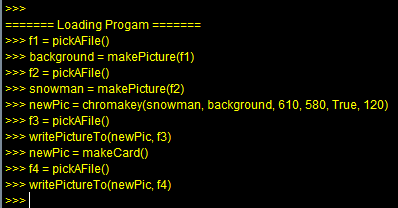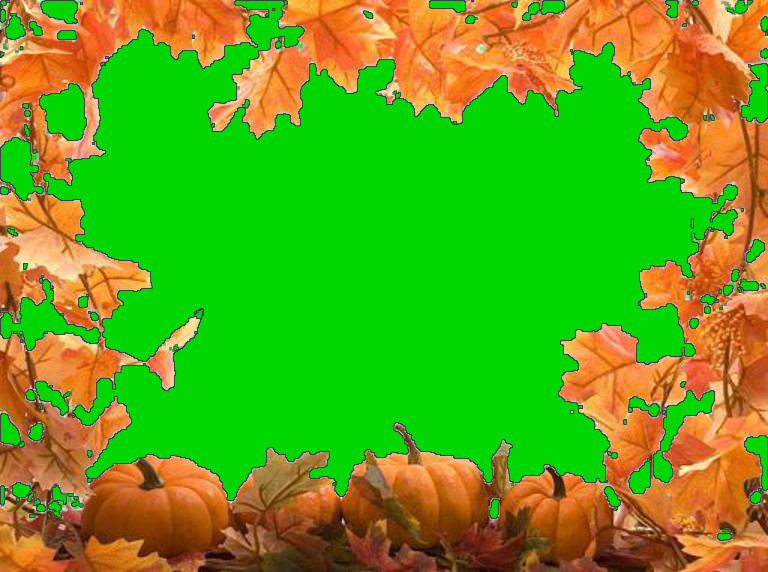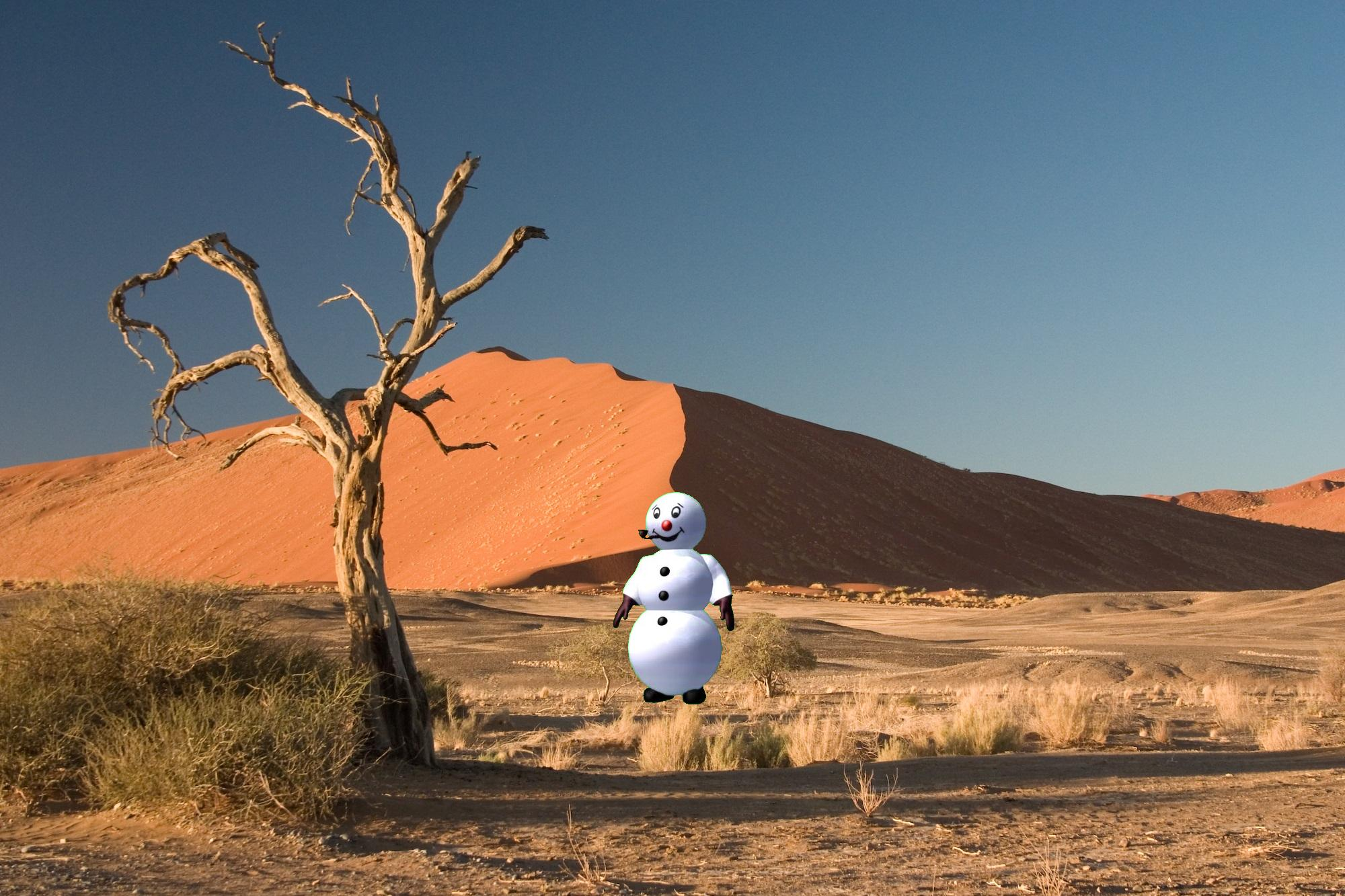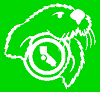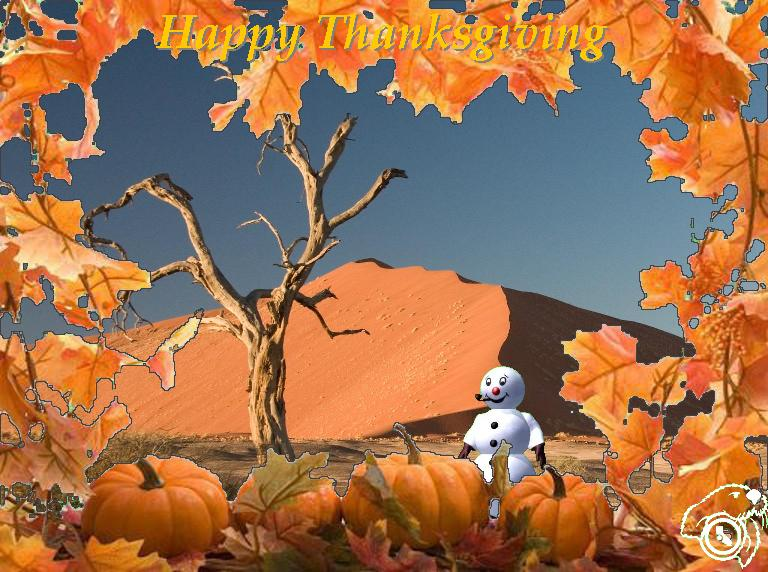Bonus: Advanced Image Processing Technique # CST205 Bonus - Programmer: John Lester pic = makePicture(pickAFile()) show(pic) def betterBnW(pic = pic): pixels = getPixels(pic) for pix in pixels: r = getRed(pix) g = getGreen(pix) b = getBlue(pix) intensity = (r * 0.299 + g * 0.587 + b * 0.114) setRed(pix, intensity) setGreen(pix, intensity) setBlue(pix, intensity) repaint(pic) return pic def lineDrawing(pic = pic): pic = betterBnW(pic) threshold = 32 for x in range(0, getWidth(pic)): for y in range(0, getHeight(pic)): pix = getPixel(pic, x, y) lum = getColor(pix) if y < getHeight(pic)-1: bpix = getPixel(pic, x, y+1) blum = getColor(bpix) if x < getWidth(pic)-1: rpix = getPixel(pic, x+1, y) rlum = getColor(rpix) if distance(lum, blum) > threshold and distance(lum, rlum) > threshold: setColor(pix, black) else: setColor(pix, white) repaint(pic) return pic pic = lineDrawing(pic) Just load it.